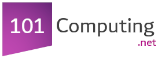## Welcome to our Binary Logic Activity page for A Level students.

By completing the following activities you will practise your binary coding skills (Denary to Binary conversions, binary addition, logic gates diagrams, truth tables and Karnaugh maps).

 Denary to Binary Conversions Binary to Denary Conversions Hexadecimal to Denary Conversions Denary to Hexadecimal Conversions Hexadecimal to Binary Conversions Binary to Hexadecimal Conversions Binary Additions Binary Subtractions Binary Shifts Negative Numbers (Sign Magnitude) Negative Numbers (Two's Complement) Binary Additions & Subtractions using Two's Complement representation Floating Points Binary Masks Logic Gates Diagrams (Find the boolean expression) Logic Gates Diagrams (Create the logic gates diagram) Truth Tables Karnaugh Maps